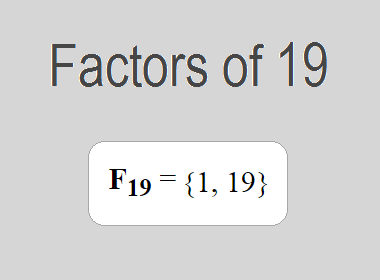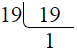# Factors of 19The factors of 19 are 1 and 19 i.e. F19 = {1, 19}. The factors of 19 are all the numbers that can divide 19 without leaving a remainder. 19 is a prime number, so it is divisible by 1 and 19 only.

We can check if these numbers are factors of 19 by dividing 19 by each of them. If the result is a whole number, then the number is a factor of 19. Let's do this for each of the numbers listed above:

·        1 is a factor of 19 because 19 divided by 1 is 19.

·        19 is a factor of 19 because 19 divided by 19 is 1.

## Properties of the Factors of 19

The factors of 19 have some interesting properties. One of the properties is that the sum of the factors of 19 is equal to 20. We can see this by adding all the factors of 19 together:

1 + 19 = 20

Another property of the factors of 19 is that the only prime factor of 19 is 19 itself.

********************

********************

## Applications of the Factors of 19

The factors of 19 have several applications in mathematics. One of the applications is in finding the highest common factor (HCF) of two or more numbers. The HCF is the largest factor that two or more numbers have in common. For example, to find the HCF of 19 and 38, we need to find the factors of both numbers and identify the largest factor they have in common. The factors of 19 are 1, and 19. The factors of 38 are 1, 2, 19, and 38. The largest factor that they have in common is 19. Therefore, the HCF of 19 and 38 is 19.

Another application of the factors of 19 is in prime factorization. Prime factorization is the process of expressing a number as the product of its prime factors. The prime factor of 19 is 19 since it is only the prime number that can divide 19 without leaving a remainder. Therefore, we can express 19 as:

19 = 19

We can do prime factorization by division method as given below,19 = 19

Since 19 is a prime number, there is no factor tree of 19.

## Conclusion

The factors of 19 are the numbers that can divide 19 without leaving a remainder. The factors of 19 are 1, and 19. The factors of 19 have some interesting properties, such as having a sum of 20. The factors of 19 have several applications in mathematics, such as finding the highest common factor and prime factorization.